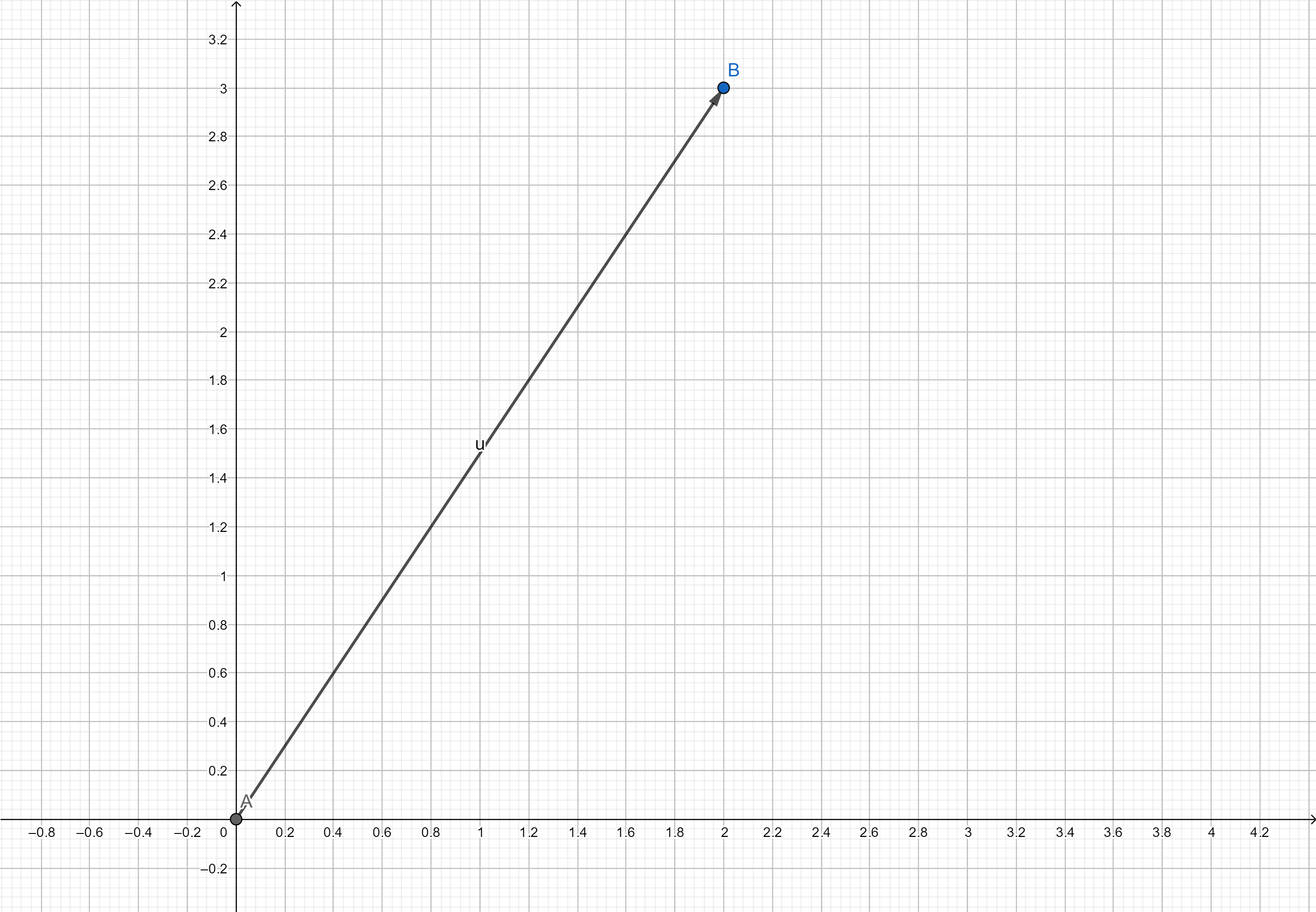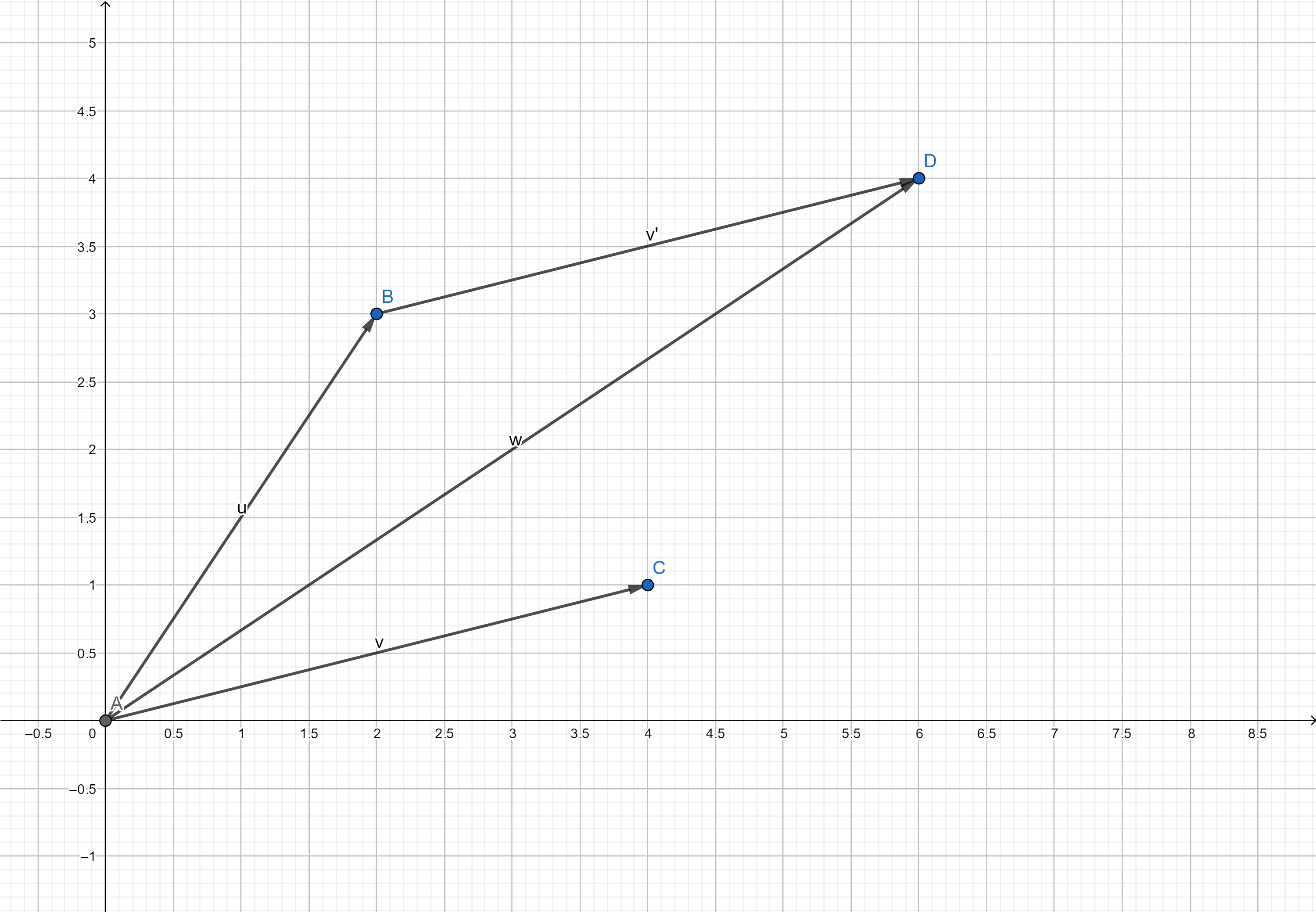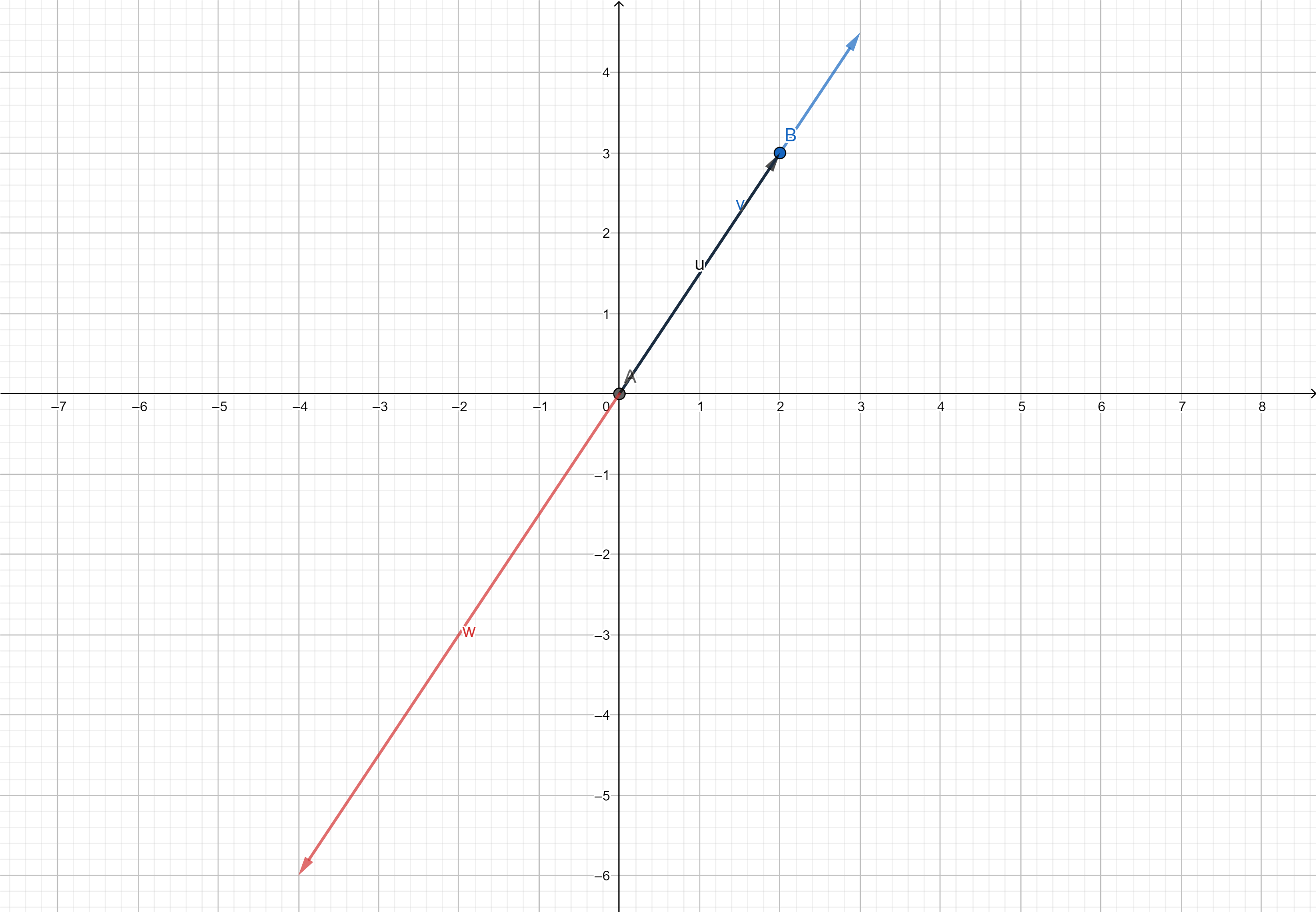向量(vector)

2019.10.4

表示方式

• 几何表示：具有方向的线段叫做有向线段，向量为有向线段（在线性代数中，通常以原点为起点）

$\vec u=\begin{bmatrix}2 \\3 \\\end{bmatrix}$ 表示为图中箭头• 坐标表示：有序的数字列表

​ 如上二维向量代表由原点指向 (a,b) 的箭头，也就是从原点在x轴移动a“步”,再在y轴移动b“步”。多维同理

基础运算

• 三角形法则

将一个向量的起点移到另一个向量的终点，这两个向量的和为后者的起点指向前者的终点 ，多个向量同理$\vec u+\vec v=\vec w$

向量数乘(vector multiplication by a number)